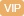| APP | 校园号 | 客服 客服热线：400-863-9889

400-863-9889A．π B．2π C．2 D．1
【考点】三角函数的周期性【专题】转化思想；综合法；三角函数的图象与性质．
【分析】圆C的标准方程为
 x2 a2
+
 y2 b2
=1
ab＞），由椭圆的一顶点恰在物线x2=8y的准线y=-2上得-b-2，解得．又
 c a
=
 3
2
，a=b+c2，立解得即可．

 3
=（x-2），椭圆的方程联化为(14k2)x28k(
 3
2k)x
+4(
 3
-2k)2
-16=0利用根与系数关系、算公式即可得出．
【解答】解：椭圆C标准方程为
 x2 a2
+
 y2 b2
=1
（a＞＞0），

a=4，c=2
 3

y-
 3
=(x-2)
x2+4y2=16

 3
k（x-2），

-8(-2k-
 3
)
1+4k2
=
8k(2+
 3
)
1+4k2

∴-b=2，解b=2．

 3
-2)x
+4(
 3
-2k)2
16=0，
 c a
=
 3
2
，2=b+c2，
∵APQ=∠BP，PA，PB的斜率为相数，

 x2 16
+
 y2 4
=1

∴x12=
8(2k-
 3
)
1+4k2

∴直线AB斜率为值
 3
6
【点评】本题考查了椭圆的标准方程其性质、直线与圆相交问题转化程立可得数的关系斜率计算公、直线方程，考查了推力与能力，属于难题．

0/0